# Construct BST from level order traversal

In this article, we are going to see how to create a BST from its level order traversal?
Submitted by Radib Kar, on October 14, 2020

In this article, we are going to see how we can construct a BST from a level order traversal? Of course, the level order traversal needs to be a valid one.

For example, say the level order traversal is: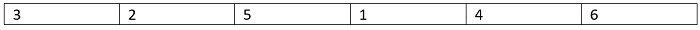Since it';s a valid BST level order traversal we can simply insert each of the nodes one by one as we did in my article on inserting in a BST.

The algorithm for insertion would be:

2. If the key to insert is less than the current value then move to the left part
3. If the key to insert is greater than the current value then move to the right part

Let's do the dry run and see how it creates the BST from the level order traversal.

First, create the root from the very first element of the level order traversal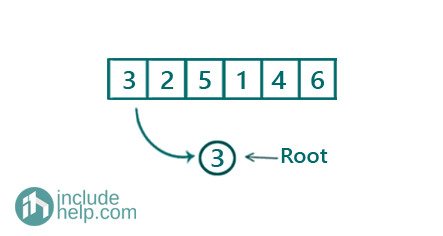Now insert the next element 2 and that will go towards left of 3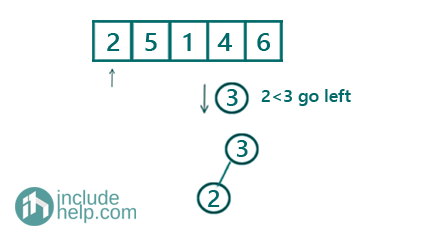After inserting 3, we need to insert 5 and that we will insert at the right subtree of 3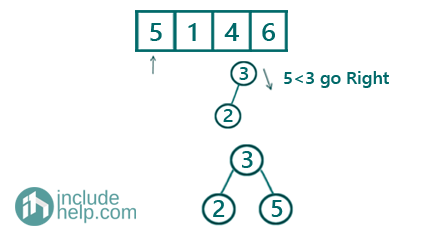Now, insert 1 like below: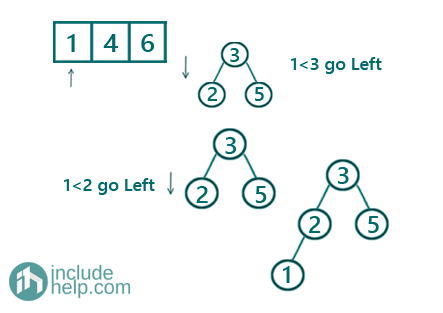Now insert 4 as below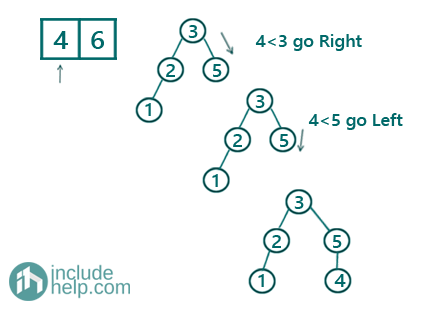And then lastly 6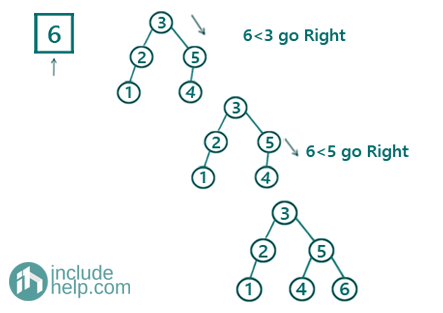So the final BST constructed is: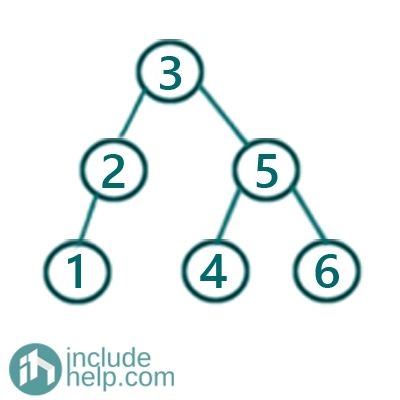Below is the C++ implementation of the above idea.

```#include <bits/stdc++.h>
using namespace std;

// tree node is defined
class TreeNode {
public:
int val;
TreeNode* left;
TreeNode* right;
TreeNode(int data)
{
val = data;
left = NULL;
right = NULL;
}
};

void inorder(TreeNode* root)
{
if (!root)
return;
inorder(root->left);
cout << root->val << " ";
inorder(root->right);
}

//recursive function to construct the tree from
//level order traversal
TreeNode* constructBSTRecur(TreeNode* root, int data)
{
if (root == NULL)
return new TreeNode(data);
//if data value is less than root go towards left
if (root->val > data)
root->left = constructBSTRecur(root->left, data);
//if data value is more than root go towards right
if (root->val < data)
root->right = constructBSTRecur(root->right, data);

return root;
}

//function to construct tree from level order traversal
TreeNode* constructBst(vector<int>& arr, int n)
{
TreeNode* root = NULL;
//loop through each element one by one
for (int i = 0; i < n; i++) {
root = constructBSTRecur(root, arr[i]);
}
return root;
}

int main()
{
//below is the level order traversal of the example tree
vector<int> arr{ 3, 2, 5, 1, 4, 6 };

cout << "Creating BST from the level order traversal\n";

TreeNode* root = constructBst(arr, arr.size());

cout << "BST created\n";
cout << "Inorder traversal of the BST is:\n";
inorder(root);

return 0;
}
```

Output:

```Creating BST from the level order traversal
BST created
Inorder traversal of the BST is:
1 2 3 4 5 6
```